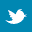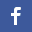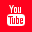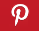Completely Solved C, C++ Programs Assignment.Quick Search Database Videos Tutorials Ebooks Forums FAQ Aboutus Household Industrial Manufacturing Service Shopping Transportation### Signal and System:Solved MATLAB Assignment I

 Filed Under: MATLAB,Signal and System

Signal and System:Solved MATLAB Assignment I
Problem 1 [A1P1]: Plotting of a unit step starting at t=2 and going to right.
Problem 2[A1P2]: Plotting of a unit impulse at t=-3.5
Problem 3[A1P3]: Plotting of a ramp of slope 2 going backward.
Problem 4[A1P4]: Generating of a 1 cycle of a sine wave
Problem 5[A1P5]: Plotting of a step of height 3 starting at t=3 and going backward to t=-α
Problem 6[A1P6]: Plotting of a signal starting at t=1 and increases linearly and constant there after.
Problem 7[A1P7]: Plotting of a stair step signal
Problem 8[A1P8]: Plotting of a ramp starting at t=2 and going downward with a slope of -3.
Problem 9[A1P9]: Plotting of a unit parabola function
Program 10[A1P10]: Plotting of a sinewave frequency 0.5 Hz sampled at 0.2 sec interval.
Problem 11[A1P11]: Plotting of a unit step sequence sampled at 1 sec interval.
Problem 12[A1P12]: Plotting of a exponential signal.
Problem 13[A1P13]: Plotting of a discrete time sinisodial signal.
Problem 14[A1P14]: Adding of two discrete time sinosodial sequence and plotting of resultant signal.